Atmos. Chem. Phys., 21, 16203–16217, 2021
https://doi.org/10.5194/acp-21-16203-2021
Atmos. Chem. Phys., 21, 16203–16217, 2021
https://doi.org/10.5194/acp-21-16203-2021

Research article 04 Nov 2021

Research article | 04 Nov 2021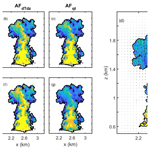# Revisiting adiabatic fraction estimations in cumulus clouds: high-resolution simulations with a passive tracer

Revisiting adiabatic fraction estimations in cumulus clouds: high-resolution simulations with a passive tracer
Eshkol Eytan1, Ilan Koren1, Orit Altaratz1, Mark Pinsky2, and Alexander Khain2 Eshkol Eytan et al.
• 1Department of Earth and Planetary Science, Weizmann Institute of Science, Rehovot, Israel
• 2Institute of Earth Science, Hebrew University, Jerusalem, Israel

Correspondence: Ilan Koren (ilan.koren@weizmann.ac.il) and Alexander Khain (alexander.khain@mail.huji.ac.il)

Abstract

The process of mixing in warm convective clouds and its effects on microphysics are crucial for an accurate description of cloud fields, weather, and climate. Still, they remain open questions in the field of cloud physics. Adiabatic regions in the cloud could be considered non-mixed areas and therefore serve as an important reference to mixing. For this reason, the adiabatic fraction (AF) is an important parameter that estimates the mixing level in the cloud in a simple way. Here, we test different methods of AF calculations using high-resolution (10 m) simulations of isolated warm cumulus clouds. The calculated AFs are compared with a normalized concentration of a passive tracer, which is a measure of dilution by mixing. This comparison enables the examination of how well the AF parameter can determine mixing effects and the estimation of the accuracy of different approaches used to calculate it. Comparison of three different methods to derive AF, with the passive tracer, shows that one method is much more robust than the others. Moreover, this method's equation structure also allows for the isolation of different assumptions that are often practiced when calculating AF such as vertical profiles, cloud-base height, and the linearity of AF with height. The use of a detailed spectral bin microphysics scheme allows an accurate description of the supersaturation field and demonstrates that the accuracy of the saturation adjustment assumption depends on aerosol concentration, leading to an underestimation of AF in pristine environments.

Share
1 Introduction

The AF is determined as

$\begin{array}{}\text{(1)}& \mathrm{AF}=\frac{\mathrm{LWC}}{{\mathrm{LWC}}_{\mathrm{ad}}},\end{array}$

where LWC is the liquid water content (g m−3) at a specific location, and LWCad is the theoretical liquid water content that a parcel would have if it was lifted adiabatically from the cloud base to a specific height. The definition of LWCad is not consistent in the literature; many studies define LWCad using the moist adiabatic lapse rate as derived by , with an inherent saturation adjustment assumption (i.e., $S\left(t,z\right)\approx \mathrm{0}$). This definition considers LWCad to be the maximal potential of LWC. This maximal value does not describe the true potential because it ignores the fact that the potential of LWCad is limited by the condensation efficiency. Saturation adjustment assumes that the total amount of water vapor that exceeds the concentration for saturation will condense instantaneously. Such an assumption ignores the relaxation time for condensation that determines the condensation efficiency and depends on the available surface area of the droplets. Cases of clouds with high supersaturation values can occur in clouds with low droplet concentrations (i.e., a low surface-area-to-volume ratio) or very strong updrafts. The various approaches for AF calculations differ in the way by which they calculate the reference LWCad. The values of LWCad can be obtained using parcel modeling or direct calculations, which can be performed in a bulk approach (e.g., using conservation of energy or water mass of all phases) or by using analytical thermodynamic considerations . The different methods are detailed in Sect. 2.3.

AF is commonly used to study the effects of mixing on clouds’ microphysical structure. Observations and numerical modeling have used AF to show the effects of mixing on the effective radius profile in cloud fields. Conditioning aircraft measurements of cumulus and stratiform clouds according to AF was used to examine the effects of mixing on the width of droplet size distribution (DSD; ). AF is also commonly used in mixing diagram analyses for determination of mixing types . Additionally, it is used to calibrate in situ aircraft measurements . Some studies approximated AF by normalizing LWC by the maximal measured value at a given height (LWCmax; ). However, some clouds may not contain adiabatic regions, or their adiabatic pockets may not be sampled; thus, the normalization of in situ measurement data by the maximal value might lead to an overestimation of AF, as ${\mathrm{LWC}}_{max}\le {\mathrm{LWC}}_{\mathrm{ad}}$. Accurate estimation of AF requires knowledge of the humidity and temperature profiles and of cloud-base height (as shown below in Sect. 2.3), which are obtained in various ways in field measurements. While the humidity and temperature profiles can be obtained by radiosondes, aircraft profiling trajectories, or remote sensing, the cloud-base height can be estimated using calculation of the lifting condensation level (LCL), lidar or ceilometer measurements, or direct sampling according to visual identification from an aircraft. The supersaturation profile, which is a nonlinear function of the humidity and temperature profiles, cannot be measured in the field at a suitable precision to the best of our knowledge. The different techniques by which the data were acquired will determine the resolution and precision, thus affecting the best choice of method to calculate AF.

In this study, we evaluated the accuracy of different methods and assumptions for AF estimation by simulating several single warm cumulus clouds in high-resolution (10 m) using different aerosol concentrations. The dynamic model is coupled to a spectral bin microphysics model for explicit representation of the microphysical processes and the resulting supersaturation field. The high resolution allows us to solve the turbulent fluxes in more detail and reduces the model dependence on sub-grid parameterizations, which improves mixing representation. Moreover, the small grid spacing enables better detection of local maxima in the 3D field (e.g., LWC, supersaturation, updraft). We confront AF with the sub-cloud layer tracer that represents the level of dilution by mixing. The simplicity and importance of AF make it applicable in many different data sets of both modeling and measurements. Since every observational data set will have different limitations (or models; e.g., varying schemes and resolutions), it is impossible for this paper to suggest one general solution to all (i.e., one algorithm of AF). This study uses a simple framework of a single cloud, while solving many of the interior complexities that affect AF, to suggest some tools for calculations of AF and to present the limitations one might encounter while doing so. External complexities such as advection, wind shear, surface fluxes, and variations of aerosols will add complexity to the cloud system but are not expected to change the nature of AF (only its resulting distribution).

Details about the model and the tracer are provided in Sect. 2.1 and 2.2, respectively. In Sect. 2.3 we derive analytical equations for LWCad and present the different assumptions that can be made for its calculations. In the “Results and discussion” section, we compare three different AF calculation methods (equations) to the sub-cloud layer tracer (Sect. 3.1). In Sect. 3.2 we show the effects of different assumptions on the accuracy of AF calculation, and in Sect. 3.3 we quantitate the accuracy of the various assumptions by sampling numerous cloudy points in space and along the clouds' lifetime, providing a larger data set for statistics.

2 Methods

## 2.1 Model description

The clouds were simulated using the System for Atmospheric Modelling (SAM; ) coupled with the Hebrew University Spectral Bin Microphysical scheme (SBM; ). SAM is a nonhydrostatic, inelastic model with cyclic boundary conditions in the horizontal direction. Sub-grid turbulence parameterization was performed using a 1.5-closure scheme. Analysis by shows that turbulent motions in this design obey the $-\frac{\mathrm{5}}{\mathrm{3}}$ law. To avoid the effects of the cloud on itself via the cyclic boundaries, we chose the domain size to be 5.12 km, which is much larger than the cloud scale (∼800 m diameter). The horizontal resolution was set to 10 m, and the vertical resolution was set to 10 m up to 3 km and 50 m for the last kilometer (maximal cloud top is 2 km). The time resolution was 0.5 s. Initial vertical profiles of water vapor mixing ratio and potential temperature (inversion at 1500–2000 m), as well as constant large-scale forcing and surface fluxes, were taken from the BOMEX case study . The horizontal background wind was set to zero and aerosols were distributed only below cloud base (600 m). The cloud was simulated for 1 h and was initialized by a perturbation of 0.1 K in the center of the domain, with a horizontal radius of 500 m and a vertical radius of 100 m (from the surface). The perturbation decays to zero as a cosine square function of $\mathrm{0} from the center to the edge of the radius, and random noise is added . The SBM is based on solving kinetic equations for size distribution functions of water drops and aerosol. Both size distributions are defined on a doubling mass grid containing 33 bins. The drop radii range between 2 µm and 3.2 mm. The size of aerosols serving as cloud condensational nuclei (CCN) ranges between 0.005 and 2 µm. Following and , the size distribution of the aerosols was represented by a sum of three lognormal distributions describing fine-, accumulation-, and coarse-mode aerosols, typical for the maritime boundary layer. Three clouds with different aerosol concentrations (Na) were simulated with Na= 5, 50, and 500 cm−3.

## 2.2 Passive tracer setup

For quantification of the dilution level of the cloud, we used a passive tracer that disperses in space and time by advection and turbulent diffusion that is set according to the sub-grid scheme. The tracer is uniformly distributed in the sub-cloud layer from the surface up to 600 m (mean cloud base). Throughout the simulation, the measured concentration is normalized by the sub-cloud initial concentration; therefore, a concentration equal to unity indicates no dilution. Figure A1 in the Appendix shows three snapshots: the tracer's initial spatial distribution, its distribution and values at the time of the cloud's maximal development (33 min), and its distribution and values at the end of the simulation after 55 min.

Although AF has been used in many studies over the years, there are different methods for calculation of LWCad, which are often not well defined in the literature (details of the calculations are often missing). The value of LWCad can be calculated in different ways that differ by method, assumptions, and practical implementations. In this section, we present three commonly used methods for AF calculation and the following assumptions that can be made.

The equation for supersaturation (S) for an adiabatically ascending parcel is given by as follows.

$\begin{array}{}\text{(2)}& \begin{array}{rl}& \frac{\mathrm{1}}{\left(S+\mathrm{1}\right)}\frac{\mathrm{d}S}{\mathrm{d}t}={A}_{\mathrm{1}}w-{A}_{\mathrm{2}}\frac{\mathrm{dLWC}}{\mathrm{d}t},\phantom{\rule{0.25em}{0ex}}\phantom{\rule{0.25em}{0ex}}\text{i.e.,}\\ & \frac{\mathrm{dlog}\left(S+\mathrm{1}\right)}{\mathrm{d}t}={A}_{\mathrm{1}}w-{A}_{\mathrm{2}}\frac{\mathrm{dLWC}}{\mathrm{d}t}\end{array}\end{array}$

$\begin{array}{}\text{(2a)}& {A}_{\mathrm{1}}=\frac{g}{T}\left(\frac{{L}_{w}}{{c}_{p}{R}_{\mathrm{v}}T}-\frac{\mathrm{1}}{{R}_{\mathrm{a}}}\right)\text{(2b)}& {A}_{\mathrm{2}}=\frac{\mathrm{1}}{{\mathit{\rho }}_{\mathrm{v}}}+\frac{{L}_{w}^{\mathrm{2}}}{{c}_{p}{R}_{\mathrm{v}}{T}^{\mathrm{2}}{\mathit{\rho }}_{\mathrm{d}}}\end{array}$

Here, w is the updraft velocity, and A1 and A2 are thermodynamics parameters, which depend on temperature and water vapor mixing ratios that vary with altitude.

Equation (2) is obtained by differentiating $S=\frac{\left(e-{e}_{\mathrm{s}}\right)}{{e}_{\mathrm{s}}}$ and using a quasi-hydrostatic approximation that is valid for updrafts weaker than 10 $\frac{m}{s}$. e is the water vapor partial pressure, es is the saturated water vapor partial pressure over liquid, g is the gravity acceleration, T is temperature, Lw is the latent heat of evaporation, cp is the heat capacity of air under constant pressure, Rv and Ra are the gas constants of water vapor and dry air, respectively, and ρv and ρd are the density of water vapor and dry air, respectively.

The first term on the right-hand side (RHS) of Eq. (2) is the source of S by adiabatic cooling, and the second term is the sink of S due to water vapor loss and latent heat release by condensation. Since Eq. (2) does not include effects of mixing, the value of LWC equals LWCad. Considering the changes in S in the vertical direction only and transforming the time domain to vertical coordinates using w leads to

$\begin{array}{}\text{(3)}& w\frac{\mathrm{dlog}\left(S+\mathrm{1}\right)}{\mathrm{d}z}={A}_{\mathrm{1}}w-w{A}_{\mathrm{2}}\frac{{\mathrm{dLWC}}_{\mathrm{ad}}}{\mathrm{d}z},\end{array}$

and the LWC in an adiabatic parcel is

$\begin{array}{}\text{(4)}& {\mathrm{LWC}}_{\mathrm{ad}}\left(z\right)=\underset{\mathrm{0}}{\overset{z}{\int }}\frac{{A}_{\mathrm{1}}\left({z}^{\prime }\right)}{{A}_{\mathrm{2}}\left({z}^{\prime }\right)}\mathrm{d}{z}^{\prime }-\underset{\mathrm{0}}{\overset{z}{\int }}\frac{\mathrm{1}}{{A}_{\mathrm{2}}\left({z}^{\prime }\right)}\frac{\mathrm{dlog}\left(S+\mathrm{1}\right)}{\mathrm{d}{z}^{\prime }}\mathrm{d}{z}^{\prime },\end{array}$

where z=0 at cloud base.

One can see that LWCad is not only a function of z, but also depends on temperature and humidity (via parameters A1 and A2), as well as on vertical velocity and aerosols through the supersaturation term. When S≪1, Eq. (4) can be simplified to the following.

$\begin{array}{}\text{(5)}& {\mathrm{LWC}}_{\mathrm{ad}}\left(z\right)=\underset{\mathrm{0}}{\overset{z}{\int }}\frac{{A}_{\mathrm{1}}\left({z}^{\prime }\right)}{{A}_{\mathrm{2}}\left({z}^{\prime }\right)}\mathrm{d}{z}^{\prime }-\underset{\mathrm{0}}{\overset{z}{\int }}\frac{\mathrm{1}}{{A}_{\mathrm{2}}\left({z}^{\prime }\right)}\frac{\mathrm{d}S}{\mathrm{d}{z}^{\prime }}\mathrm{d}{z}^{\prime }\end{array}$

Equation (5) shows that in regions where S increases with height (e.g., near cloud base or in pristine environments), LWCad will be smaller than its maximal value because some amount of water vapor in excess of supersaturation remains in the gas phase. At the exception to these cases, the S term is small compared to the first term on the RHS of Eq. (5). Neglecting the term which includes the supersaturation, we can write the following.

$\begin{array}{}\text{(6)}& {\mathrm{LWC}}_{\mathrm{ad}}\left(z\right)\approx \underset{\mathrm{0}}{\overset{z}{\int }}\frac{{A}_{\mathrm{1}}\left({z}^{\prime }\right)}{{A}_{\mathrm{2}}\left({z}^{\prime }\right)}\mathrm{d}{z}^{\prime }\end{array}$

Taking $\frac{{A}_{\mathrm{1}}}{{A}_{\mathrm{2}}}$ as a constant leads to the well-known linear LWCad profile, which is the first assumption to be examined in this study (Sect. 3.2.1).

Two alternative approaches can be used to calculate LWCad. One is using the total water mixing ratio ${q}_{\mathrm{t}}={q}_{\mathrm{l}}+{q}_{\mathrm{v}}$ (g kg−1), which is a conservative value in moist adiabatic processes. qv is the water vapor mixing ratio and ql is the liquid water mixing ratio. At cloud base ${q}_{l}={q}_{{l}_{\mathrm{0}}}\approx \mathrm{0}$, so ${q}_{{t}_{\mathrm{0}}}={q}_{{v}_{\mathrm{0}}}$. For undiluted parcels ${q}_{{t}_{\mathrm{0}}}={q}_{\mathrm{t}}\left(z\right)$, and at any altitude above cloud base ${q}_{\mathrm{t}}\left(z\right)={q}_{\mathrm{l}}\left(z\right)+{q}_{\mathrm{v}}\left(z\right)$. Assuming saturation adjustment (i.e., S(z)=0) means that qv=qvs, where qvs is the water vapor mixing ratio in saturation that can be calculated according to the Clausius–Clapeyron equation. LWCad can then be defined using ql as follows.

$\begin{array}{}\text{(7)}& {\mathrm{LWC}}_{\mathrm{ad}}\left(z\right)=\left[{q}_{{\mathrm{vs}}_{\mathrm{0}}}-{q}_{\mathrm{vs}}\left(z\right)\right]{\mathit{\rho }}_{\mathrm{d}}\left(z\right)\end{array}$

Such an approach was used by (Hermann E. Gerber, personal communication, 2020).

The third approach is to use the conservation of moist static energy (h; ), where $h={L}_{w}{q}_{\mathrm{v}}+{c}_{p}T+gz$ (J kg−1). Differentiating h with respect to z, conserving it with height ($\frac{\mathrm{d}h}{\mathrm{d}z}=\mathrm{0}$), assuming water mass conservation (i.e., $\mathrm{d}{q}_{\mathrm{v}}=-\mathrm{d}{q}_{\mathrm{l}}$, and multiplying by ρd gives the following.

$\begin{array}{}\text{(8)}& {\mathrm{LWC}}_{\mathrm{ad}}\left(z\right)=\frac{{c}_{p}}{{L}_{w}}\underset{\mathrm{0}}{\overset{z}{\int }}{\mathit{\rho }}_{\mathrm{d}}\left({z}^{\prime }\right)\left(\frac{g}{{c}_{p}}+\frac{\mathrm{d}T}{\mathrm{d}{z}^{\prime }}\right)\mathrm{d}{z}^{\prime }\end{array}$

Equation (8) shows that the difference between the lapse rate of an adiabatic parcel and the dry adiabatic lapse rate (${\mathrm{\Gamma }}_{\mathrm{d}}=\frac{g}{{c}_{p}}$) is due to condensation and can thus be translated into LWCad. We note here that this method avoids the use of saturation adjustments.

3 Results and discussion

## 3.1 Comparison between the three methods

In this work, we analyze the growth and mature stages of shallow cumulus clouds, before obtaining considerable sedimentation flux. Shallow Cu lifetime in general is short; hence, the radiative heating by the weak absorption of solar radiation or cooling by thermal radiation emittance can be neglected. Therefore, we did not calculate radiation transfer during the simulation. Neglecting sedimentation and radiation allows us to use AF as a measure of mixing. The Lagrangian equations presented above were solved from the outputs of the Eulerian model using the assumption that on a timescale of ∼5 min the thermodynamic profiles in the cloud are fixed during growth and mature stages. It implies that the profiles of temperature and humidity can be used to predict the conditions to which a parcel in the cloud base would be exposed as it ascends. The most accurate way to consider profiles of temperature (T(z)) and specific humidity (qv(z)) for LWCad calculations is to obtain them from the undiluted core of the cloud, where qv is maximal and T is warmer due to release of latent heat. If there is a perfect undiluted adiabatic core, its AF value is equal to 1, and it will coincide with the maximum normalized value of the tracer (Tr); thus, Tr can be used as a first-order approximation for AF. Figure 1 shows cross sections of Tr and the three different methods for calculating AF (see list below) when it reached its maximal height and mass (33 min). The sensitivity of the methods to the choice of profiles is tested in Fig. 1 by calculating each method twice: first with accurate “least diluted” profiles and second by approximating the adiabatic (undiluted) profiles, using the points with the highest updraft values at each level.

The methods are denoted as follows.

1. AFref is calculated according to Eq. (6) using the in-cloud profiles of A1 and A2. This method for AF calculation will be used as the reference method from here on (reasoning for this choice is provided below).

2. AFdTdz is calculated according to Eq. (8).

3. AFqt is calculated according to Eq. (7).

The accurate estimations of the adiabatic vertical profiles of T and qv were obtained here by averaging the values of those parameters in the voxels containing the highest 1 % Tr values at each altitude (minimal threshold that was used was Tr = 0.67 in the highest levels of the cloud), and the results are presented in Fig. 1a–c. The cross section of Tr is provided in Fig. 1d.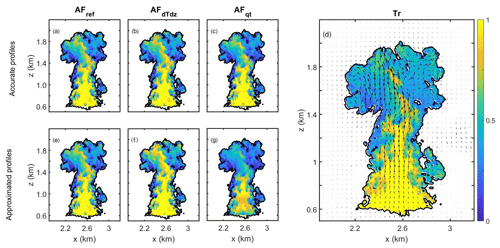Figure 1Cross sections of the tracer and the three AF methods presented for a cloud with a 500 cm−3 aerosol concentration at the time of its maximal top height. The AFs in the upper row were calculated using accurate adiabatic profiles of T and qv. Profiles in the bottom row were approximated using high updrafts. (a) The method for calculating AF used as a reference (AFref) by solving Eq. (6). (b) Same as (a) for AFdTdz using Eq. (8). (c) Same as (a) for AFqt using Eq. (7). (d) Normalized concentration of the sub-cloud layer tracer (Tr). (e) Same as (a) for approximated profiles. (f) Same as (b) using approximated profiles. (g) Same as (c) using approximated profiles.

The vertical profiles of T and qv that were used in Fig. 1a–c (based on the simulated Tr) can also be calculated using the maximal values of LWC or updraft. It is hard to obtain these types of profiles from in situ measurements that do not contain the theoretical tracer or the full 3D distribution of the cloud variables. Thus, for the sake of simplicity and in order for the methods presented in this paper to be comparable to measurements, we approximated the profiles by averaging the values in the voxels with the highest 5 % updraft values at each altitude. This methodology was used to estimate the T and qv profiles throughout this study. It is shown that AFref remains almost similar when using either the approximated or accurate profiles. On the other hand, AFqt and AFdTdz exhibit some underestimation and overestimation compared to the accurate profiles, respectively. These differences are explained in detail below, and tests of sensitivity to the chosen profiles according to different thresholds on Tr values are presented in the Appendix for all three methods (Fig. A2). Figure 2 presents the differences between each AF method when using the approximated profiles and the Tr values (as shown in Fig. 1d). The apparently good agreement between AFref and Tr, as presented in Fig. 1e, is more closely examined in Fig. 2a, where differences are detected. In the first 100 m above cloud base the AFs experience non-realistic, non-homogenous values due to the inhomogeneity of the cloud base (in agreement with results of ). Moreover, the values of LWC and LWCad near the cloud base are small; hence, their ratio exhibits high sensitivity even when differences from the reference are minor (chosen according to the highest updraft). For the sake of comparison with Tr, the points near cloud base with AF > 1 were set to 1. Determination of the cloud-base height was achieved using the vertical profile of the cloud horizontal cross-sectional area. All clouds exhibited a local maximum in the cross-sectional area around 600 m during their growing stage. Aiming to choose the cloud base as a level that can represent the cloud with “enough” cloudy voxels, we chose to define it as the height above the level of initial condensation, in which the area covers 90 % of the local maximal area. Changing the criteria threshold from 90 % to 33 % can decrease the cloud-base height by up to 30 m. This definition, using the 90 % criteria, was found to be stable for all simulations during the growing stage of the clouds and is considered optimal for AF calculations since it maintains optimal agreement between AFref and Tr in the regions of high values. When comparing the cross sections of Tr with each AF presented in Fig. 1 (more than 100 m above cloud base), one can see that the AFs decrease toward the cloud edge faster than Tr (Tr > AF; see Fig. 2a). This is because AF is also affected by evaporation, and not only dilution, as in the case of Tr (i.e., mechanical mixing). The opposite is observed in higher levels in slightly diluted regions, where $\mathrm{Tr}<{\mathrm{AF}}_{\mathrm{ref}}<\mathrm{1}$. These regions represent a more complex difference between AF and Tr, which is also caused by condensation and/or evaporation. Tr can change only due to mechanical mixing and is hence almost a one-directional process; once the parcel is diluted, it has low probability to restore its initial Tr concentration. This means that Tr has a memory of the mixing history, unlike AF that can be influenced by source and sink processes. A parcel can regain liquid water after a mixing event if supersaturation is reached again at a later stage. Moreover, the parcel's condensation rate can be different from that predicted by the adiabatic parcel model because its droplet size distributions have changed and the local profiles of supersaturation can be very different from the ones of the core. This means that a parcel in the margins of the cloud can be diluted, decreasing both Tr and LWC (AF), but later, if the parcel gains vertical velocity and supersaturation, it might condense water at a rate that is larger than in the core. This will compensate for the LWC loss (keeping Tr the same, while increasing AF; i.e., $\frac{\mathrm{dLWC}}{\mathrm{d}z}>\frac{{\mathrm{dLWC}}_{\mathrm{ad}}}{\mathrm{d}z}$). The toroidal vortex seems to be a mechanism that drives such conditions. In Fig. 2a we show red regions of AF > Tr, which are voxels of relatively strong updrafts and are part of the flow pattern of the toroidal vortex (for an elaborated discussion about the vortex see ). Using the velocity field, the regions of AF > Tr can be tracked back in time (back trajectory) to their earlier location, where the toroidal vortex entrains environmental air. Those parcels that mix with entrained air are first diluted and then flow upward driven by the flow in the toroidal vortex. These diluted parcels with low droplet concentration and high vertical velocity create high supersaturation values (higher than the values in the core for the same altitude). Hence, they condense water at a higher rate, which leads to a local increase in AF with altitude. The phenomenon of rapid growth of droplets in an updraft following an entrainment event was suggested as a mechanism for rain initiation . Correlations of the red regions (where AF > Tr) with strong updrafts (as part of the toroidal vortex), high supersaturation values, and low droplet concentration were found for different time steps and different cloud simulations.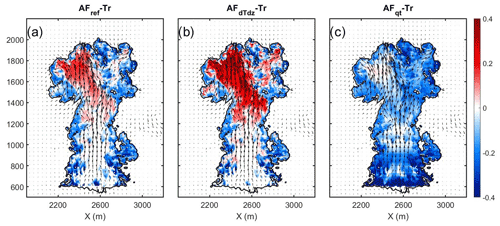Figure 2Vertical cross sections for the differences between various AF methods, using approximated profiles (Fig. 1e–g), and the tracer (Fig. 1d). (a) Difference between AFref and Tr (Fig. 1e minus Fig. 1d). (b) Same as (a) for AFdtdz. (c) Same as (a) for AFqt.

Figure 1f shows the cross section of AFdTdz values, and Fig. 2b shows its difference from Tr. Here, very good agreement with AFref is observed, although AFdTdz with the approximated profiles is slightly larger in the sub-adiabatic regions at higher levels (i.e., having smaller values of LWCad(z)). This is explained by the fact that AFdTdz considers the difference between $\frac{\mathrm{d}T}{\mathrm{d}z}$ and the dry lapse rate as a consequence of condensation and uses it to define LWCad. Diluted parcels are colder than the adiabatic core because they were mixed with colder environmental air and may have experienced evaporation. This difference between absolutely adiabatic and slightly diluted parcels increases with height as the parcel is aging. For these reasons, using diluted voxels to estimate the adiabatic profiles will lead to a larger temperature gradient (more negative) that is closer to the dry lapse rate, falsely inferring less condensation and biasing LWCad toward smaller values. The arguments above explain the difference between Fig. 1a–b where AFdTdzAFref and Fig. 1e–f where AFdTdz> AFref. These findings suggest that AFref is less sensitive to the choice of adiabatic profiles because it is constrained by two free parameters (T and qv) rather than only T. The final method, AFqt, is shown to be very sensitive to the choice of profiles, as presented in Fig. 1c and g. The deviation from Tr in Fig. 2c demonstrates a substantial underestimation of AFqt due to a similar argument as discussed above for AFdTdz. Using a slightly diluted parcel, with a smaller qv or qvs compared to the core, is expected to falsely infer more condensation and larger LWCad. The bias is stronger for this method because it depends on qv (or its estimation as qvs(T)). The simplicity of this method is also its downfall; since qv is an order of magnitude larger than ql (liquid mixing ratio), small errors can cause significant effects when estimating LWCad using only qv. Another disadvantage of AFqt is that it is commonly used with the saturation adjustment assumption (i.e., S≈0) by estimating qv to be qvs. This assumption can lead to underestimation of AFqt in conditions of a pristine environment (low aerosol concentrations) as explained in detail in Sect. 3.2.3. The results of this section suggest that the analytical solution for AFref using Eq. (5) is a more accurate and stable method to calculate AF, as it shows similarity to Tr and robustness for different choices of vertical profiles. We also note that profiles of T and qv are often obtained from the environment (see Sect. 3.2.2). This will lead to substantial errors when using AFdTdz or AFqt, which showed sensitivity to the choice of profiles. Furthermore, Eq. (5) allows isolating different assumptions, such as linearity, with height and saturation adjustments.

## 3.2 Testing the effects of assumptions made when calculating AF

Next, we examine the effects of several commonly used assumptions on AFref calculation in order to estimate their impact. Although the magnitude of the difference that should be considered significant depends on the application, we define a considerable difference here as 0.1, which is 10 % of the maximal LWCad.

The approaches that will be examined next are the following:

1. AFlinear using Eq. (6) and keeping $\frac{A\mathrm{1}}{A\mathrm{2}}$ constant from the cloud base;

2. AFenv using the sounding (environmental) profiles in Eq. (6);

3. AFs including the supersaturation term using Eq. (5);

4. AF+50 using Eq. (6) but estimating the cloud-base height to be 50 m higher; and

5. AF−50 using Eq. (6) but estimating the cloud-base height to be 50 m lower.

Each approach will be compared with the reference approach (AFref; Eq. 6). This method is not the most accurate one (method AFs using Eq. 5 is), but since it is the base for all other examined assumptions, using it allows us to isolate and examine each of the assumptions separately.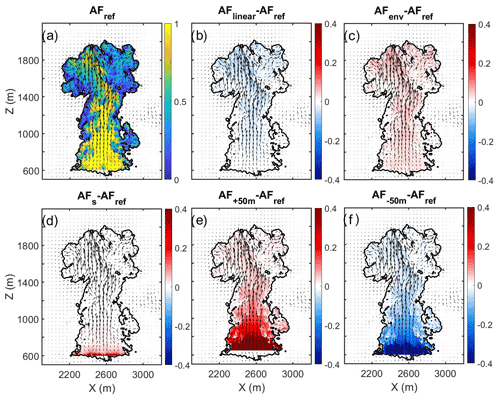Figure 3Vertical cross sections of the differences between the various assumptions used when calculating AF. (a) The method of calculating AF that is used as the reference (AFref; as presented in Fig. 1e). (b) AFref subtracted from an AF that is linear from cloud base (AFlinear). (c) AFref subtracted from AFenv, calculated using sounding (environmental) profiles. (d) AFref subtracted from AFs, which considers the supersaturation term (Eq. 5). (e) The error in AF caused by overestimating cloud base by +50 m. (f) Same as (e) with a −50 m error.

AFlinear is a very common method based on the assumption that LWCad is linear with height (i.e., neglecting the dependence of $\frac{A\mathrm{1}}{A\mathrm{2}}$ on temperature and humidity). This implies that $\frac{A\mathrm{1}}{A\mathrm{2}}$ can be used as a constant based on the known values at the cloud base . Note that the derivation of AF using leads to the equation of $\frac{A\mathrm{1}}{A\mathrm{2}}$ at cloud base. Indeed, small negative differences from the nonlinear AFref (i.e., underestimation) are observed in Fig. 3b when using $\frac{A\mathrm{1}}{A\mathrm{2}}$ as a constant from the cloud base. derived such a solution for stratocumulus clouds and noted that the error using this method would increase for deeper clouds. On the same note, argued that the linear assumption is valid for shallow clouds (depth of up to 200 hPa, ≈2 km). This emphasizes that the usage of the AFlinear method is restricted to shallow clouds and should be used with care or avoided altogether for deeper clouds.

The changes in the growth rate of LWCad ($\frac{A\mathrm{1}}{A\mathrm{2}}$ in Eq. 6) with height, which can lead to deviation from AFlinear (or AFenv, discussed next), occur mostly due to changes in A2. This is because A1 (Eq. 2a), which is a parameter in the term for cooling by ascent, depends only on temperature and exhibits a negligible change in the case of our shallow clouds. A2 (Eq. 2b), which relates to the S sink term (by condensation), depends on T and qv and increases with height. Figure 4 demonstrates the sensitivity of A2 to qv and T by presenting the differences in the profiles of A2 from the true profile (calculated using the original qv and T profiles) and when keeping T or qv as a constant (with the value set at cloud base). The true profile of A2 (in blue) shows an increase with height, as mentioned earlier. One can see that the A2 profile with a constant T (red curve) is very similar to the true profile. On the other hand, the profile's gradient decreases significantly when using qv as a constant (yellow line). This demonstrates that depletion of water vapor in higher levels of the cloud is the major factor that impacts A2 values (see the inverse relation to water vapor mixing ratio in Eq. 2b) and the deviation of LWCad from its linear relations.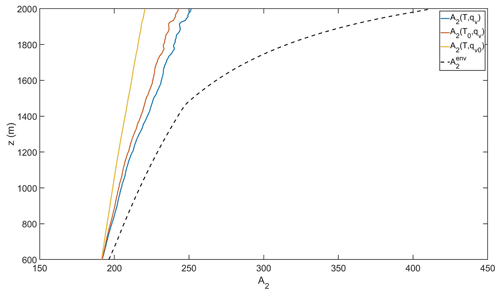Figure 4The sensitivity of the A2 profile to temperature and humidity. The growth rate of LWCad depends on the ratio of $\frac{A\mathrm{1}}{A\mathrm{2}}$ (see Eq. 6). LWCad changes with height depend mostly on A2, since A1 exhibits little sensitivity. The blue curve is the true profile of A2. Red and yellow A2 profiles are calculated using constant temperature and humidity, respectively, and the dashed black line is the profile obtained using the environmental sounding.

### 3.2.2LWCad using sounding profiles (environmental profiles)

The advantage of using environmental profiles is that they can be obtained from sounding data and can be considered constant reference values for an ensemble of clouds (the whole cloud field). Such an application can have large errors in cases in which the T and qv profiles in the cloud's core and the environment exhibit large differences (e.g., penetration of the cloud into the inversion layer or into higher levels of the atmosphere). The profile of A2 when calculated using the environmental profiles is given as a dashed black line in Fig. 4. It shows that the environmental A2 profile values are larger than the profiles in the core of the cloud (especially in the inversion layer above 1500 m, where qv decreases fast). The larger A2 values lead to a smaller LWCad (A2 is in the denominator in Eq. 6) and hence to an overestimation of LWCenv, as seen in Fig. 3c. The small overestimation witnessed in our case (trade wind cumulus in Barbados) will probably be greater for deeper clouds, wherein the gradients between the core and the environment are larger.

### 3.2.3 The role of the supersaturation term in conditions of low aerosol concentrations

Considering the profiles of T and qv is necessary if one wishes to dismiss the saturation adjustment assumption (i.e., $\frac{\mathrm{d}S}{\mathrm{d}z}\approx \mathrm{0}$). This assumption is almost inherent in most previous works that we know of. The supersaturation can be significantly greater than zero in regions of high updrafts and/or small droplet concentrations (for example, in the first tens of meters above cloud base and in pristine environments). Thus, if one wants to achieve accuracy near cloud base or compare different clouds under different aerosol loading conditions (e.g., studying aerosol effects on cloud mixing), one has to use AFs as calculated by Eq. (5). The second term in Eq. (5), referred to here as the S term, depends on the vertical profile of S. It is worth noting that S profiles are available only in modeling studies, since in situ measurements of S cannot reach the desired accuracy, as far as we know. Figure 3d demonstrates that for cases in which the cloud develops under conditions of high aerosol concentrations (Na= 500 cm−3), neglecting the S term introduces a negligible underestimation of AF near the cloud base (AFs>AFref). However, this is not the case for cleaner environments (lower Na). Neglecting the S term means assuming that the parcel condenses all of the excess water vapor that forms as it ascends and cools. This overlooks the limited condensation efficiency in pristine environments that prevents a full consumption of water vapor by the drops and lets S increase (i.e., $\frac{\mathrm{d}S}{\mathrm{d}z}$ and the second term are larger than zero). To evaluate this effect, we simulated two additional clouds in a cleaner environment with lower aerosol concentrations (Na= 50 and 5 cm−3). Before analyzing these simulations, we first had to make sure that there is no significant sedimentation in the clouds at this stage. Sedimentation creates liquid water loss and downdrafts, which violate the adiabatic assumption and lead to a deviation of AF from Tr. For the example examined in this section, we used Tr to ensure that sedimentation can be neglected in the time steps we chose for comparison (timing of maximal cloud-top height of each cloud). Figure A3 in the Appendix shows vertical cross sections of AFref and Tr for the cloud simulations with Na= 50 and 5 cm−3 at the time steps used for this example. There is good agreement between AFref and Tr for Na= 50 cm−3 at 33 min and Na = 5 cm−3 at 31 min. This is not the case for Na= 5 cm−3 after 40 min when the cloud precipitates and sedimentation can no longer be neglected. In Fig. A3 we observe regions in the clouds with large differences between Tr and AFref values.

In Fig. 5, we present the deviation of AFs (when including the saturation term) from AFref for the three different simulations. Figure 5a (same as Fig. 3d) shows that the deviation is negligible for high Na. The deviation increases and spreads to higher levels when Na is smaller (Fig. 5b–c). Under pristine conditions (Na= 5 cm−3, Fig. 5c), the underestimation of AFref spreads throughout the entire cloud column. The profiles of S are depicted in Fig. 5d for all cases, presented as the mean S of the voxels with the highest 5 % updraft in each level. Figure 5d shows that saturation adjustment is a reasonable approximation for AF calculations in polluted clouds because the S profile is smaller and, more importantly, almost constant. The gradient of the S profile of the Na= 50 cm−3 case is also positive far from the cloud base (at 1000–1400 m). It does not introduce very large errors to AFref (as can be seen in Fig. 5b) because the gradient is relatively small, and thus the second term in Eq. (5) is negligible compared to the first term. Near cloud base, the first term in Eq. (5) is small, and thus even a relatively small gradient in S can be significant and lead to a large difference between AFref and AFs.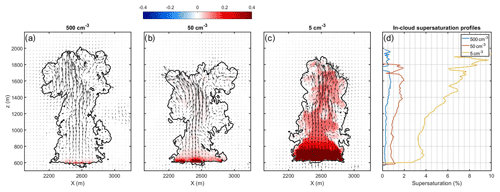Figure 5Microphysical effects on AF. The droplet concentration (i.e., aerosols) affects AF through the profile of supersaturation inside the cloud. Panels (a)(c) present cross sections of AFs (with the supersaturation term) minus AFref. (a) For high Na of 500 cm−3 (as in Fig. 3d). (b) For 50 cm−3 at 33 min. (c) For 5 cm−3 at 31 min. (d) In-cloud supersaturation profiles.

### 3.2.4 AF sensitivity to cloud-base heights

Last, we tested how sensitive AF calculations are to the errors in the estimation of the cloud-base height. Figure 3e–f show the deviation from AFref when having an error of ±50 m in cloud-base height. When overestimating (underestimating) cloud-base height, as in Fig. 3e (Fig. 3f), the estimated LWCad is smaller (larger) and AF is larger (smaller). These results demonstrate that such small errors in a parameter that is often taken for granted can introduce large errors in adiabaticity estimation. For example, the lifting condensation level (LCL), which is often used to approximate cloud-base height, can be obtained from a tephigram or calculated by several proposed analytical equations. Calculating LCL from surface conditions, as suggested by , , and , approximates cloud-base height to be 515, 550, or 525 m, respectively. These approximations are lower than the height that was found to be optimal for AF calculations using the sub-cloud layer tracer (≈600 m, depending on simulation time and cloud properties). Additionally, LCL is known to be an underestimation of cloud-base height when the convective parcel is driven by perturbation in temperature. In such a case, the perturbation reduces the parcel's relative humidity, and therefore the parcel starts the condensation at a higher altitude (above the LCL). This can cause an overestimation of LWCad and an underestimation of AF. The opposite will occur when the convection is driven by a humidity fluctuation . We note that most in situ measurements and spaceborne remote sensing observations lack the tools required to measure the cloud-base height and usually estimate it based on LCL.

## 3.3 Mean differences between the assumptions with time

So far, we have examined the AF calculations at one time step of the time of maximum development of the clouds (∼33 min). The robustness of the results is tested by estimating the deviation of each method from AFref over height and as a function of time along the cloud's lifetime. Since the deviations are more pronounced in regions of high AF, for this analysis we chose to consider only the cloudy regions with AFref>0.5. Note that these sub-adiabatic regions are important and highly debated. Figure 6 shows the mean deviation for a cloud with Na= 500 cm−3. Here, we observe that the statistics along the cloud's lifetime agree with the instantaneous qualitative pictures presented in Fig. 3. As demonstrated in Fig. 6a, the linear assumption (AFlinear) underestimates AF for altitudes above cloud base. AFenv, using the environmental profiles (Fig. 6b), exhibits a small overestimation, which becomes significant near the cloud top at the inversion layer. Here, we define a significant difference as larger than 0.1 in absolute value (marked by the black contour). Considering the S term in the polluted case does not have a considerable effect (Fig. 6c). Errors in the estimations of cloud base by 50 m lead to relatively large errors in AF- up to 1000 m (Fig. 6d–e). Repeating the same analysis for the clean case (with Na= 5 cm−3) gives similar results for all methods but AFs (see Fig. 7c), which supports the argument made in Sect. 3.2.3. The time series is shorter in this case because sedimentation starts after 33 min. It seems that the observed differences between the AF calculation methods do not change with time during the growth stage of a particular cloud for both pristine and polluted conditions.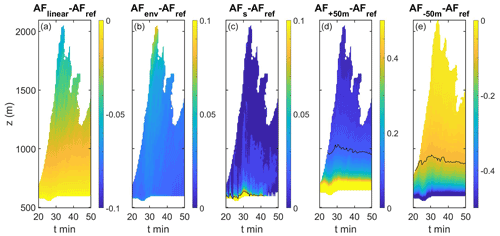Figure 6Mean differences in AFs vs. time and altitude for a polluted cloud. The horizontal average of the difference of each assumption from AFref for regions with AFref>0.5 is presented. (a) The difference between the linear method AFlinear and AFref. (b) Same as (a) for AFenv. (c) Same as (a) for AFs. (d) The error in AF produced by an error of +50 m in cloud-base height. (e) Same as (d) for −50 m. The black contour marks the 0.1 or −0.1 deviation. Note the different scales for the different panels.

4 Summary and conclusions

An accurate calculation of the adiabatic fraction (AF) is crucial in two main aspects. First, it can promote a high-resolution measure of the mixing state of sampled parcels. This may advance the research of mixing processes in shallow clouds and their effects, which remain open questions in the field of cloud physics. Second, it can allow mapping the occurrence and extent of adiabatic regions in shallow clouds (which is still under debate). Since adiabatic processes are simpler to predict it is highly beneficial to assume them in remote sensing retrieval algorithms and cloud parameterizations in weather and climate models. Answering these questions can improve our process-level understanding, in situ measurements, and remote sensing retrievals. This will improve models' representation of shallow convection and may reduce the magnitude of the shallow clouds' contribution to the uncertainty in weather and climate models.

This study used high-resolution (10 m) simulations of isolated trade wind cumulus clouds that solved the turbulent flow down to scales that are rarely achieved. This enables a better representation of mixing and relaxes the dependency on sub-grid parameterization schemes. A sub-cloud layer's passive tracer (Tr), which is an accurate measure of mechanical mixing, was added to the simulations and used as a reference. This model configuration enabled better control of AF and the complex processes that it represents, as well as giving a theoretical framework that allowed testing the accuracy of different approaches that are commonly used to calculate adiabatic fraction (AF). Three different derivation methods of AF (Eqs. 5, 7, 8) were compared with the tracer. While the most robust method (Eq. 5) depends on both temperature and humidity profiles, the other two methods (Eq. 7, 8) depend on one variable (humidity or temperature, respectively). The method that is based only on humidity (Eq. 7) exhibited weaker agreement with Tr. Comparing AF with Tr also shows that some regions in the cloud emphasized the important differences between AF and Tr. While Tr follows the complex flow in the cloud and records all mixing events, AF is based on a one-dimensional model whose reference lies in the core. For that reason, AF cannot describe processes that occur in the margins. Moreover, condensation that occurs after a mixing event can delete records of earlier evaporation or dilution events. As an example, the toroidal vortex drives entrainment events followed by updrafts, which cause some parcels to experience dilution and evaporation (decrease in Tr and AF), followed by condensation that increases AF. The analytical structure of the reference method (Eq. 5) also allows us to isolate different assumptions and evaluate their accuracy. The important findings and their implications are as follows: assuming a linear profile of LWCad or using the sounding profiles of temperature and humidity instead of the in-cloud profile produces small errors at higher levels of shallow clouds (∼2 km). The small error in AF for shallow clouds obtained using environmental profiles suggests that it can be used as a constant reference for all clouds in the field.

The saturation adjustment assumption was integrated into the calculation of AF in most previous studies. Testing this assumption on clouds that develop in different environmental conditions (with different aerosol concentrations) revealed that it can lead to underestimation of AF. A simulation of a cloud in a pristine environment (Na= 5 cm−3) yielded high supersaturation values (compared to the polluted case) and led to an underestimation of AF when assuming saturation adjustment (i.e., $\frac{\mathrm{d}S}{\mathrm{d}z}\approx \mathrm{0}$). This means that comparing clouds' mixing under different aerosol loading when using the saturation adjustment assumption may neglect some of the microphysical effects on clouds' dynamics, mixing in particular.

AF was found to be sensitive to errors of ±50 m in the estimated cloud-base height, especially in the first few hundred meters above cloud base. Determining cloud-base height is challenging for aircraft in situ measurements and is often obtained by estimating the lifting condensation level (LCL) from a tephigram or analytical solutions. The three analytical solutions that were tested here differed by 45 m and underestimated the cloud-base height that was optimal for AF calculations. Underestimation of the cloud-base height can lead to a larger LWCad and thus to the underestimation of AF. This can lead to an underestimation of the extent of adiabatic regions in shallow clouds. Accurate estimation of AF near the cloud base is challenging because these levels include a ratio of two small numbers (LWC and LWCad) and are not homogenous as mostly assumed. Moreover, the calculation of LWCad in these levels exhibits high sensitivity to the determination of cloud base and the representative supersaturation profile.

All simulations demonstrated the existence of an adiabatic core (i.e., high values that are close to 1 for both AF and Tr) up until the cloud top. While the core is wide at the lower parts of the cloud, it narrows and breaks down to smaller fragments at higher levels. The extent and frequency of adiabatic parcels in different levels of the cloud will be assessed in a subsequent study.

And in short, here are the main points to consider when practically calculating AF.

• 1.

Calculations of AF will be most robust when using Eq. (5) (or Eq. 6 in polluted conditions), including the linear assumption when it is valid .

• 2.

When using AF for studies of aerosol–cloud interactions by comparing different parameters conditioned by AF, one cannot make the saturation adjustment assumption as it underestimates AF in pristine conditions and can bias the results.

• 3.

AF is most sensitive to the definition of cloud-base height. Thus, it is important to make sure that the chosen value represents the investigated cloud or clouds well at the altitude in which most parcels start to condense water.

• 4.

AF in deep convective clouds is prone to many large errors, and the uncertainty of the calculations is hard to assess, mainly in relation to the following.

• a.

AF is based on a quasi-hydrostatic equation, which is valid for updrafts smaller than 10 m s−1.

• b.

Supersaturation in clouds with strong updrafts can increase, leading to underestimations of AF when the S term is neglected.

• c.

Sedimentation of particles from higher levels of deep clouds can increase LWC in the lower levels and lead to an overestimation of AF.

• d.

The rate of change in LWCad is dominated by the parameter A2, which changes as water vapor is depleted in clouds, meaning that LWCad ceases to be linear. The large differences expected in deep clouds between the in-cloud and environmental profiles suggest that the latter are prone to large biases when used to predict AF.

Appendix A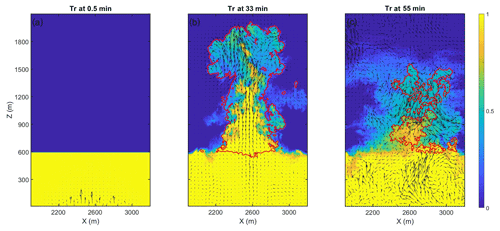Figure A1The sub-cloud layer's passive tracer. Vertical cross sections of the tracer along the x axis in the middle of the domain. Tr is the tracer's mixing ratio normalized by its initial mixing ratio in the sub-cloud layer. (a) The initial distribution at the beginning of the simulation. (b) Distribution at the time of the cloud's maximal development (33 min). Red contours mark the cloud boundaries where the liquid mixing ratio is smaller than 0.01 g kg−1. (c) Same as (b) for the end of the simulation when the cloud is nearly completely dissipated.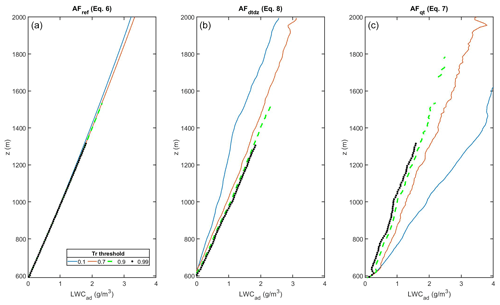Figure A2LWCad profiles of different approaches for different estimations of adiabatic profiles. LWCad(z) was calculated according to Eqs. (6, a), (8, b), and (7, c). Taken from a snapshot of a cloud with an aerosol concentration of 500 cm−3 at the time of maximal development (33 min). The temperature and humidity profiles were used by averaging all points of each layer according to a certain threshold on sub-layer tracer normalized concentration (Tr). Black dots are for nearly pure undiluted parcels with Tr > 0.99, the green dashed line is for nearly adiabatic parcels (Tr > 0.9), and red and blue curves also include slightly (Tr > 0.7) and strongly diluted (Tr > 0.1) parcels. It is shown that there are no pure adiabatic parcels above the inversion. Nevertheless, the use of slightly diluted parcels (with Tr > 0.7) in our chosen reference method (Eq. 6) does not introduce large biases to LWCad and AF accordingly.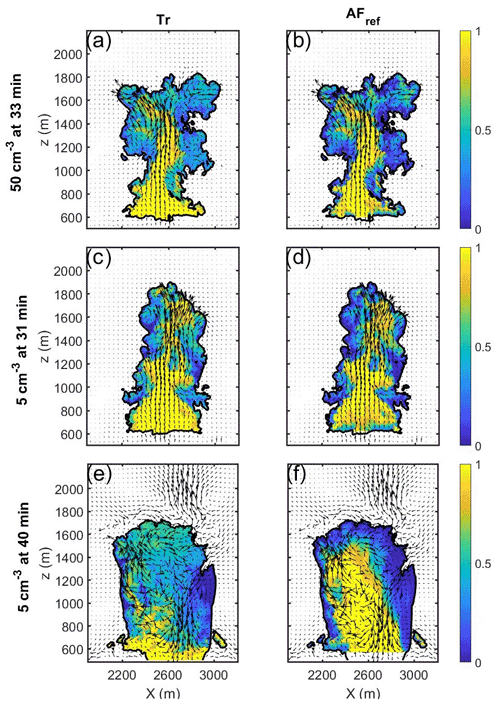Figure A3Comparison of AFref and Tr for low Na. Cross sections of AFref and Tr for lower Na simulations. (a) Tr for 50 cm−3 at 33 min. (b) Same as (a) for AFref. (c) Tr for 5 cm−3 at 31 min prior to intense sedimentation. (b) Same as (c) for AFref. (e) Tr for 5 cm−3 at 40 min during intense sedimentation. (f) Same as (e) for AFref.

Code availability

The SAM codes should be requested from Marat Khairoutdinov from the School of Marine and Atmospheric Sciences, Stony Brook University (further information can be found at http://rossby.msrc.sunysb.edu/~marat/SAM.html, last access: 2 November 2021).

Data availability

The microphysical and thermodynamical profiles used to initialize the simulation can be obtained upon request from the corresponding author.

Author contributions

EE, IK, and AK jointly conceived the principal idea. EE carried out the analysis. EE, IK, OA, AK, and MP discussed results and wrote the paper.

Competing interests

The contact author has declared that neither they nor their co-authors have any competing interests.

Disclaimer

Publisher's note: Copernicus Publications remains neutral with regard to jurisdictional claims in published maps and institutional affiliations.

Acknowledgements

Eshkol Eytan was supported by the Weizmann Institute Sustainability and Energy Research Initiative.

Financial support

This project has received funding from the European Research Council (ERC) under the European Union's Horizon 2020 research and innovation program (CloudCT, grant agreement no. 810370). Alexander Khain and Mark Pinsky were supported by grants from the Department of Energy (grant no. DE-964SC0008811) and the Israel Science Foundation (grants 2027/17 and 2635/20).

Review statement

This paper was edited by Hang Su and reviewed by two anonymous referees.

References

Albrecht, B. A.: Aerosols, cloud microphysics, and fractional cloudiness, Science, 245, 1227–1230, 1989. a

Altaratz, O., Koren, I., Reisin, T., Kostinski, A., Feingold, G., Levin, Z., and Yin, Y.: Aerosols' influence on the interplay between condensation, evaporation and rain in warm cumulus cloud, Atmos. Chem. Phys., 8, 15–24, https://doi.org/10.5194/acp-8-15-2008, 2008. a

Baker, M., Corbin, R., and Latham, J.: The influence of entrainment on the evolution of cloud droplet spectra: I. A model of inhomogeneous mixing, Q. J. Roy. Meteor. Soc., 106, 581–598, 1980. a

Bera, S.: Droplet spectral dispersion by lateral mixing process in continental deep cumulus clouds, J. Atmos. Sol.-Terr. Phy., 214, 105550, https://doi.org/10.1016/j.jastp.2021.105550, 2021. a, b

Bolton, D.: The computation of equivalent potential temperature, Mon. Weather Rev., 108, 1046–1053, 1980. a, b

Boucher, O., Randall, D., Artaxo, P., Bretherton, C., Feingold, G., Forster, P., Kerminen, V.-M., Kondo, Y., Liao, H., Lohmann, U., Rasch, P., Satheesh, S. K., Sherwood, S., Stevens, B., and Zhang, X. Y.: Clouds and aerosols, in: Climate change 2013: the physical science basis. Contribution of Working Group I to the Fifth Assessment Report of the Intergovernmental Panel on Climate Change, 571–657, Cambridge University Press, 2013. a

Brenguier, J.-L.: Parameterization of the condensation process: A theoretical approach, J. Atmos. Sci., 48, 264–282, 1991. a, b

Brenguier, J.-L., Bachalo, W. D., Chuang, P. Y., Esposito, B. M., Fugal, J., Garrett, T., Gayet, J.-F., Gerber, H., Heymsfield, A., Kokhanovsky, A., and Korolev, A.: In situ measurements of cloud and precipitation particles, Airborne Measurements for Environmental Research: Methods and Instruments, 225–301, Wiley-VCH Verlag & Co. KGaA, Weinheim, Germany, 2013. a

Ceppi, P., Brient, F., Zelinka, M. D., and Hartmann, D. L.: Cloud feedback mechanisms and their representation in global climate models, WIRES Clim. Change, 8, e465, https://doi.org/10.1002/wcc.465, 2017. a

Dagan, G., Koren, I., Altaratz, O., and Heiblum, R. H.: Time-dependent, non-monotonic response of warm convective cloud fields to changes in aerosol loading, Atmos. Chem. Phys., 17, 7435–7444, https://doi.org/10.5194/acp-17-7435-2017, 2017. a

De Rooy, W. C., Bechtold, P., Fröhlich, K., Hohenegger, C., Jonker, H., Mironov, D., Pier Siebesma, A., Teixeira, J., and Yano, J.-I.: Entrainment and detrainment in cumulus convection: An overview, Q. J. Roy. Meteor. Soc., 139, 1–19, 2013. a, b

Fan, J., Ovtchinnikov, M., Comstock, J. M., McFarlane, S. A., and Khain, A.: Ice formation in Arctic mixed-phase clouds: Insights from a 3-D cloud-resolving model with size-resolved aerosol and cloud microphysics, J. Geophys. Res.-Atmos., 114, D04205, https://doi.org/10.1029/2008JD010782, 2009. a

Freud, E., Rosenfeld, D., Andreae, M. O., Costa, A. A., and Artaxo, P.: Robust relations between CCN and the vertical evolution of cloud drop size distribution in deep convective clouds, Atmos. Chem. Phys., 8, 1661–1675, https://doi.org/10.5194/acp-8-1661-2008, 2008. a

Gerber, H.: Structure of small cumulus clouds, in: Proc. 13th Int. Conf. on Clouds and Precipitation, 105–108, 14–18 August 2000, Reno, Nevada, 2000. a

Gerber, H. E., Frick, G. M., Jensen, J. B., and Hudson, J. G.: Entrainment, mixing, and microphysics in trade-wind cumulus, J. Meteorol. Soc. Jpn. Ser. II, 86, 87–106, 2008. a, b

Hirsch, E., Koren, I., Altaratz, O., Levin, Z., and Agassi, E.: Enhanced humidity pockets originating in the mid boundary layer as a mechanism of cloud formation below the lifting condensation level, Environ. Res. Lett., 12, 024020, https://doi.org/10.1088/1748-9326/aa5ba4, 2017. a

IPCC: Climate Change 2013: The Physical Science Basis. Contribution of Working Group I to the Fifth Assessment Report of the Intergovernmental Panel on Climate Change, Cambridge University Press, Cambridge, United Kingdom and New York, NY, USA, https://doi.org/10.1017/CBO9781107415324, 2013. a

Jaenicke, R. G.: Numerical Data and Functional Relationships in Science and Technology: Volume 4, Meteorology; Subvolume B, Physical and Chemical Properties of the Air, Geophysics and Space Research, New Series, Group V, Springer-Verlag, Germany, 1988. a

Khain, A., Pokrovsky, A., Pinsky, M., Seifert, A., and Phillips, V.: Simulation of effects of atmospheric aerosols on deep turbulent convective clouds using a spectral microphysics mixed-phase cumulus cloud model. Part I: Model description and possible applications, J. Atmos. Sci., 61, 2963–2982, 2004. a

Khain, A. P. and Pinsky, M.: Physical processes in clouds and cloud modeling, Cambridge University Press, 2018. a, b

Khain, P., Heiblum, R., Blahak, U., Levi, Y., Muskatel, H., Vadislavsky, E., Altaratz, O., Koren, I., Dagan, G., Shpund, J., and Khain, A.: Parameterization of vertical profiles of governing microphysical parameters of shallow cumulus cloud ensembles using LES with bin microphysics, J. Atmos. Sci., 76, 533–560, 2019. a

Khairoutdinov, M. F. and Randall, D. A.: Cloud resolving modeling of the ARM summer 1997 IOP: Model formulation, results, uncertainties, and sensitivities, J. Atmos. Sci., 60, 607–625, 2003. a

Kim, B.-G., Miller, M. A., Schwartz, S. E., Liu, Y., and Min, Q.: The role of adiabaticity in the aerosol first indirect effect, J. Geophys. Res.-Atmos., 113, D05210, https://doi.org/10.1029/2007JD008961, 2008. a

Korolev, A. V. and Mazin, I. P.: Supersaturation of water vapor in clouds, J. Atmos. Sci., 60, 2957–2974, 2003. a

Lawrence, M. G.: The relationship between relative humidity and the dewpoint temperature in moist air: A simple conversion and applications, B. Am. Meteorol. Soc., 86, 225–234, 2005. a, b

Merk, D., Deneke, H., Pospichal, B., and Seifert, P.: Investigation of the adiabatic assumption for estimating cloud micro- and macrophysical properties from satellite and ground observations, Atmos. Chem. Phys., 16, 933–952, https://doi.org/10.5194/acp-16-933-2016, 2016. a

Nuijens, L. and Siebesma, A. P.: Boundary layer clouds and convection over subtropical oceans in our current and in a warmer climate, Current Climate Change Reports, 5, 80–94, 2019. a

Ovtchinnikov, M. and Kogan, Y. L.: An investigation of ice production mechanisms in small cumuliform clouds using a 3D model with explicit microphysics. Part I: Model description, J. Atmos. Sci., 57, 2989–3003, 2000. a

Pandithurai, G., Dipu, S., Prabha, T. V., Maheskumar, R., Kulkarni, J., and Goswami, B.: Aerosol effect on droplet spectral dispersion in warm continental cumuli, J. Geophys. Res.-Atmos., 117, D16202, https://doi.org/10.1029/2011JD016532, 2012. a

Pawlowska, H., Grabowski, W. W., and Brenguier, J.-L.: Observations of the width of cloud droplet spectra in stratocumulus, Geophys. Res. Lett., 33, L19810, https://doi.org/10.1029/2006GL026841, 2006. a

Pinsky, M., Eytan, E., Koren, I., Altaratz, O., and Khain, A.: Convective and turbulent motions in non-precipitating Cu. Part 1: Method of separation of convective and turbulent motions, J. Atmos. Sci., 78, 2307–2321, https://doi.org/10.1175/JAS-D-20-0127.1, 2021. a

Pontikis, C.: Parameterization of the droplet effective radius of warm layer clouds, Geophys. Res. Lett., 23, 2629–2632, 1996. a, b, c

Romps, D. M.: Exact expression for the lifting condensation level, J. Atmos. Sci., 74, 3891–3900, 2017. a, b

Romps, D. M. and Kuang, Z.: Do undiluted convective plumes exist in the upper tropical troposphere?, J. Atmos. Sci. 67, 468–484, 2010a. a, b

Romps, D. M. and Kuang, Z.: Nature versus nurture in shallow convection, J. Atmos. Sci., 67, 1655–1666, 2010b. a, b

Schmeissner, T., Shaw, R., Ditas, J., Stratmann, F., Wendisch, M., and Siebert, H.: Turbulent mixing in shallow trade wind cumuli: Dependence on cloud life cycle, J. Atmos. Sci., 72, 1447–1465, 2015. a, b

Sherwood, S. C., Bony, S., and Dufresne, J.-L.: Spread in model climate sensitivity traced to atmospheric convective mixing, Nature, 505, 37–42, 2014. a

Siebesma, A. P., Bretherton, C. S., Brown, A., Chlond, A., Cuxart, J., Duynkerke, P. G., Jiang, H., Khairoutdinov, M., Lewellen, D., Moeng, C.-H., and Sanchez, E.: A large eddy simulation intercomparison study of shallow cumulus convection, J. Atmos. Sci., 60, 1201–1219, 2003. a

Small, J. D., Chuang, P. Y., Feingold, G., and Jiang, H.: Can aerosol decrease cloud lifetime?, Geophys. Res. Lett., 36, L16806, https://doi.org/10.1029/2009GL038888, 2009. a

Yang, F., Shaw, R., and Xue, H.: Conditions for super-adiabatic droplet growth after entrainment mixing, Atmos. Chem. Phys., 16, 9421–9433, https://doi.org/10.5194/acp-16-9421-2016, 2016. a

Yau, M. K. and Rogers, R. R.: A short course in cloud physics, Elsevier, UK, 1996. a, b, c, d

Zelinka, M. D., Myers, T. A., McCoy, D. T., Po-Chedley, S., Caldwell, P. M., Ceppi, P., Klein, S. A., and Taylor, K. E.: Causes of higher climate sensitivity in CMIP6 models, Geophys. Res. Lett., 47, e2019GL085782, https://doi.org/10.1029/2019GL085782, 2020. a

Zhang, S., Xue, H., and Feingold, G.: Vertical profiles of droplet effective radius in shallow convective clouds, Atmos. Chem. Phys., 11, 4633–4644, https://doi.org/10.5194/acp-11-4633-2011, 2011.  a

Zhao, M. and Austin, P. H.: Life cycle of numerically simulated shallow cumulus clouds. Part II: Mixing dynamics, J. Atmos. Sci., 62, 1291–1310, 2005. a